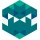Home
IT Knowledge
Inspiration
Languages
EN

# Python - find character index in string

0 points
Created by:Nataniel-Barclay
289

In this article, we would like to show you how to find a character index in string in Python.

Quick solution:

``````text = 'ABC'
index = text.find('A')

print(index)  # A``````

## Practical example

In this example, we use the String `find()` method to find indexes of the characters in a given string.

``````text = 'ABC'

indexA = text.find('A')
indexB = text.find('B')
indexZ = text.find('Z')

print(indexA)  # 0
print(indexB)  # 1
print(indexZ)  # -1``````

Output:

``````0
1
-1``````